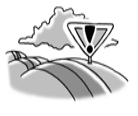### Home > PC > Chapter 2 > Lesson 2.3.4 > Problem2-116

2-116.Graph $h ( x ) = \left\{ \begin{array} { l l } { ( x - 2 ) ^ { 2 } } & { \text { for } x \geq 0 } \\ { 3 x + 4 } & { \text { for } x < 0 } \end{array} \right.$. Try this using the 2-116 eTool (Desmos).

1. Shift the graph $2$ units to the left and $3$ units down. Graph it on the same set of axes.

2. Write the equation of the new function and call it $j\left(x\right)$.

Use the eTool below to complete parts (a) and (b).
Click the link at right for the full version of the eTool: 2-116 HW eTool (Desmos)